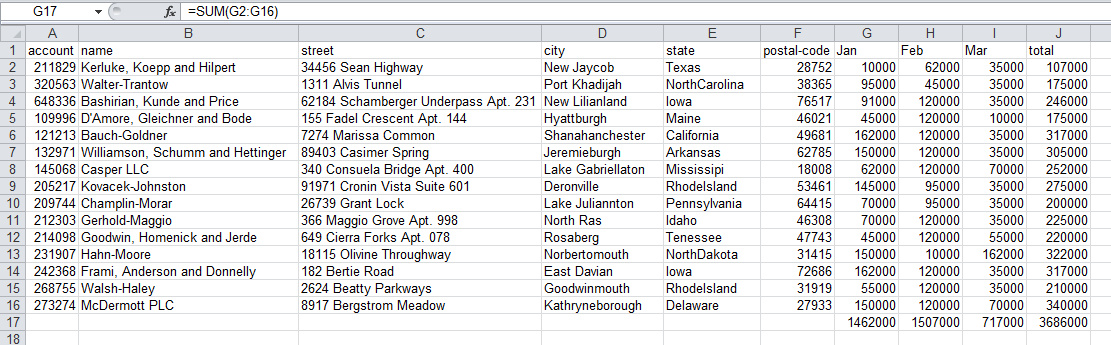﻿ 用Python的pandas框架操作Excel文件中的数据教程_python_脚本之家
python# 用Python的pandas框架操作Excel文件中的数据教程

```import pandas as pd
import numpy as np
``````df["total"] = df["Jan"] + df["Feb"] + df["Mar"]
``````df["Jan"].sum(), df["Jan"].mean(),df["Jan"].min(),df["Jan"].max()

(1462000, 97466.666666666672, 10000, 162000)
```

```sum_row=df[["Jan","Feb","Mar","total"]].sum()
sum_row

Jan   1462000
Feb   1507000
Mar    717000
total  3686000
dtype: int64
```

```df_sum=pd.DataFrame(data=sum_row).T
df_sum
``````df_sum=df_sum.reindex(columns=df.columns)
df_sum

``````df_final=df.append(df_sum,ignore_index=True)
df_final.tail()
``````from fuzzywuzzy import fuzz
from fuzzywuzzy import process
state_to_code = {"VERMONT": "VT", "GEORGIA": "GA", "IOWA": "IA", "Armed Forces Pacific": "AP", "GUAM": "GU",
"KANSAS": "KS", "FLORIDA": "FL", "AMERICAN SAMOA": "AS", "NORTH CAROLINA": "NC", "HAWAII": "HI",
"NEW YORK": "NY", "CALIFORNIA": "CA", "ALABAMA": "AL", "IDAHO": "ID", "FEDERATED STATES OF MICRONESIA": "FM",
"Armed Forces Americas": "AA", "DELAWARE": "DE", "ALASKA": "AK", "ILLINOIS": "IL",
"Armed Forces Africa": "AE", "SOUTH DAKOTA": "SD", "CONNECTICUT": "CT", "MONTANA": "MT", "MASSACHUSETTS": "MA",
"PUERTO RICO": "PR", "Armed Forces Canada": "AE", "NEW HAMPSHIRE": "NH", "MARYLAND": "MD", "NEW MEXICO": "NM",
"MISSISSIPPI": "MS", "TENNESSEE": "TN", "PALAU": "PW", "COLORADO": "CO", "Armed Forces Middle East": "AE",
"NEW JERSEY": "NJ", "UTAH": "UT", "MICHIGAN": "MI", "WEST VIRGINIA": "WV", "WASHINGTON": "WA",
"MINNESOTA": "MN", "OREGON": "OR", "VIRGINIA": "VA", "VIRGIN ISLANDS": "VI", "MARSHALL ISLANDS": "MH",
"WYOMING": "WY", "OHIO": "OH", "SOUTH CAROLINA": "SC", "INDIANA": "IN", "NEVADA": "NV", "LOUISIANA": "LA",
"NORTHERN MARIANA ISLANDS": "MP", "NEBRASKA": "NE", "ARIZONA": "AZ", "WISCONSIN": "WI", "NORTH DAKOTA": "ND",
"Armed Forces Europe": "AE", "PENNSYLVANIA": "PA", "OKLAHOMA": "OK", "KENTUCKY": "KY", "RHODE ISLAND": "RI",
"DISTRICT OF COLUMBIA": "DC", "ARKANSAS": "AR", "MISSOURI": "MO", "TEXAS": "TX", "MAINE": "ME"}
```

```process.extractOne("Minnesotta",choices=state_to_code.keys())

('MINNESOTA', 95)

process.extractOne("AlaBAMMazzz",choices=state_to_code.keys(),score_cutoff=80)
```

```def convert_state(row):
abbrev = process.extractOne(row["state"],choices=state_to_code.keys(),score_cutoff=80)
if abbrev:
return state_to_code[abbrev]
return np.nan
```

```df_final.insert(6, "abbrev", np.nan)
``````df_final['abbrev'] = df_final.apply(convert_state, axis=1)
df_final.tail()
``````df_sub=df_final[["abbrev","Jan","Feb","Mar","total"]].groupby('abbrev').sum()
df_sub

``````def money(x):
return "\${:,.0f}".format(x)

formatted_df = df_sub.applymap(money)
formatted_df
``````sum_row=df_sub[["Jan","Feb","Mar","total"]].sum()
sum_row
```

```Jan   1462000
Feb   1507000
Mar    717000
total  3686000
dtype: int64
```

```df_sub_sum=pd.DataFrame(data=sum_row).T
df_sub_sum=df_sub_sum.applymap(money)
df_sub_sum
``````final_table = formatted_df.append(df_sub_sum)
final_table
``````final_table = final_table.rename(index={0:"Total"})
final_table
```